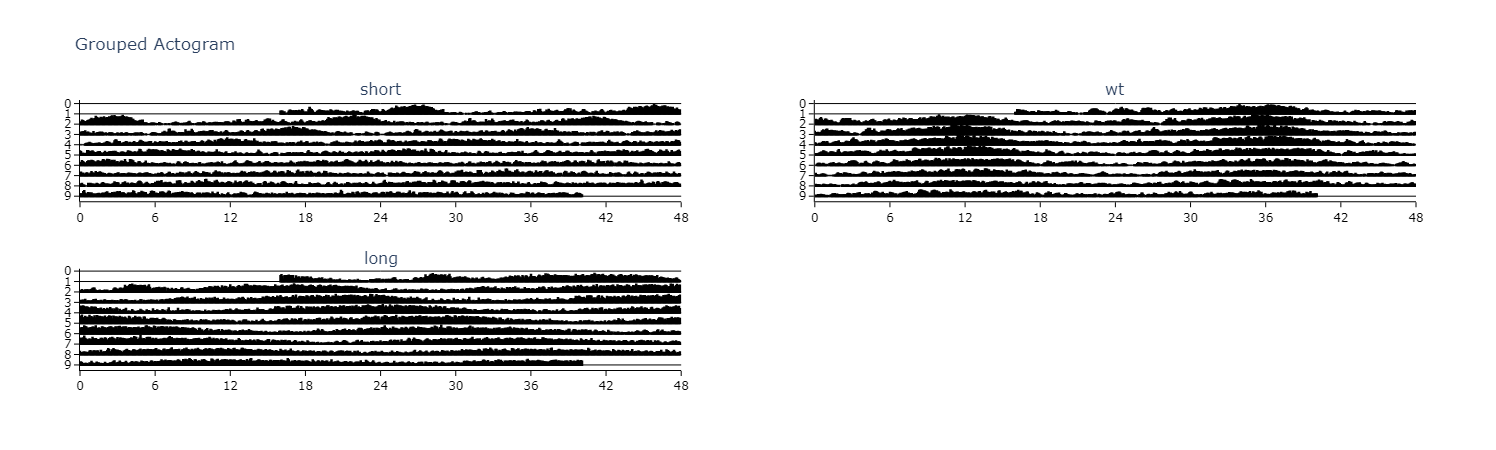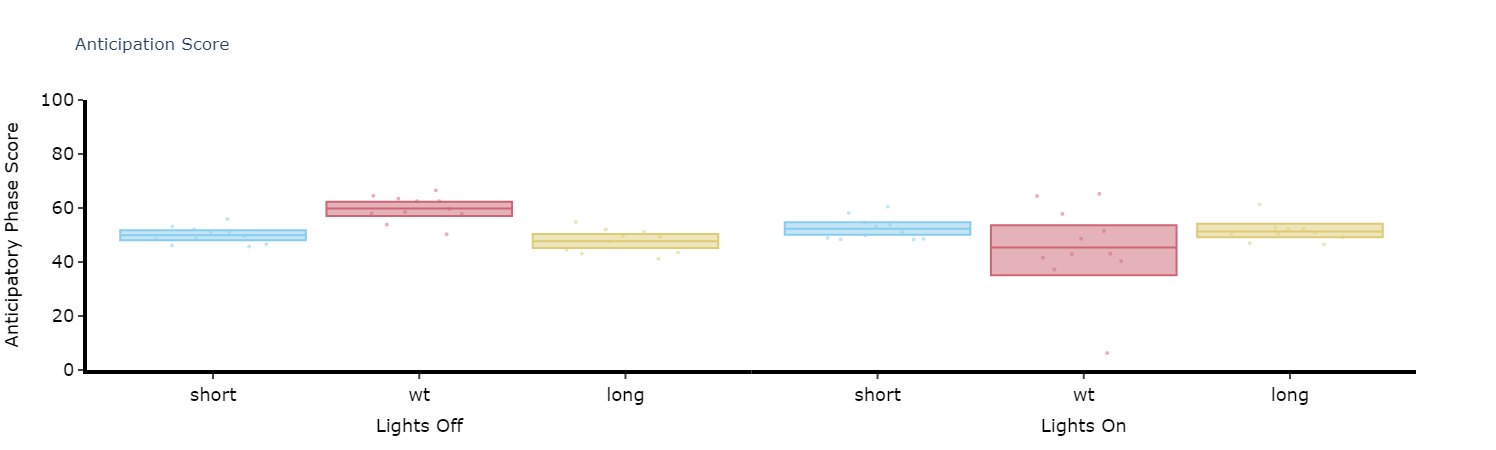The below methods and plots should give a good insight into your specimens circadian rhythm. If you think another method should be added please don't hesitate to contact us and we'll see what we can do.

Head to the circadian notebook for an interactive run through of everything below.

### Actograms

Actograms are one of the best initial methods to quickly visualise changes in the rhythm of a specimen over several days. An actogram double plots each days variable (usually movement) so you can compare each day with its previous and following day.

``````# .plot_actogram() can be used to plot indivdual specimens actograms or plot the average per group
# below we'll demonstrate a grouped example

fig = df.plot_actogram(
mov_variable = 'moving',
bin_window = 5, # the default is 5, but feel free to change it to smooth out the plot or vice versa
facet_col = 'period_group',
facet_arg = ['short', 'wt', 'long'],
title = 'Grouped Actogram')

fig.show()````````````# plot_actogram_tile will plot every specimen in your behavpy dataframe
# Be careful if your dataframe has lots of flies as you'll get a very crowded plot!
fig = df.plot_actogram_tile(
mov_variable = 'moving',
labels = None, # By default labels is None and will use the ID column for the labels.
# However if individual labels in the metadata add that column here. See the tutorial for an example
title = 'Tiled Actogram')

fig.show()
``````

### Anticipation Score

Many animals including Drosophila have peaks of activity in the morning and evening as lights turn on and off respectively. Given this daily activity the activity score looks to quantify when the specimens internal clock anticipates these moments. The score is calculated as the ratio of the final 3 hours of activity prior to lights on and off compared to the whole 6 hours prior.

``````# Simply call the plot_anticipation_score() method to get a box plot of your results
# the day length and lights on/off time is 24 and 12 respectively, but these can be changed if you're augmenting the environment

fig, stats = df.plot_anticipation_score(
mov_variable = 'moving',
facet_col = 'period_group',
facet_arg = ['short', 'wt', 'long'],
day_length = 24,
lights_off = 12,
title = 'Anticipation Score')

fig.show()``````